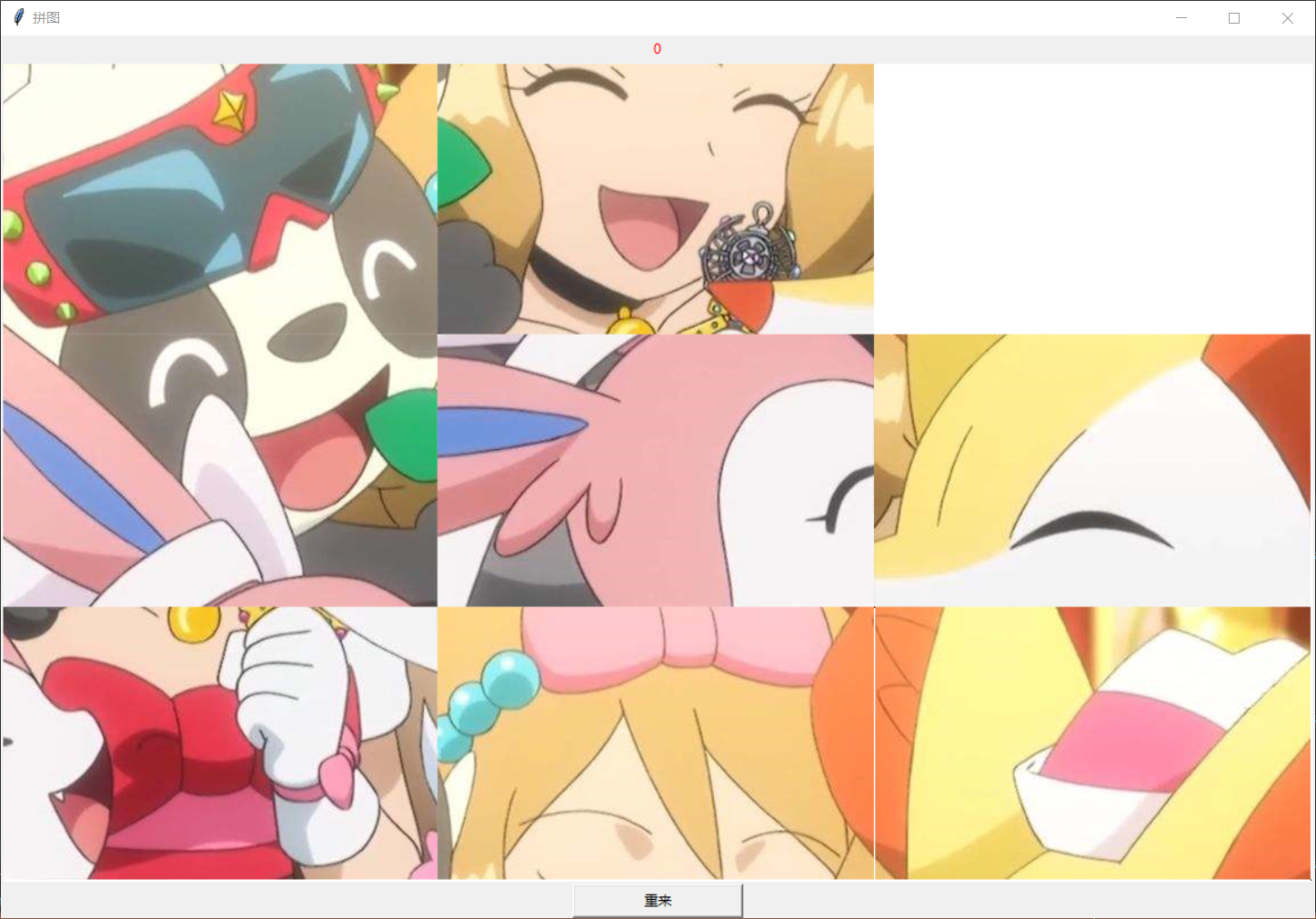# python 基于tkinter的拼图游戏

03/08 14:53

``````from tkinter import *
from tkinter.messagebox import *
import random

root =Tk('拼图')
root.title('拼图')

pics=[]      #读取外部图片并存到一个数组方阵中
for i in range(9):
filename='Serina'+str(i)+'.png'
pics.append(PhotoImage(file=filename))

Width=1152
Height=720

image_width=Width//3
image_height=Height//3

Rows=3
Cols=3

steps=0

board=[[0,1,2],
[3,4,5],
[6,7,8]]

class Square:
def __init__(self,orderID):
self.orderID=orderID
def draw(self,canvas,board_pos):
img=pics[self.orderID]
canvas.create_image(board_pos,image=img)

def init_board():
l=list(range(8))
l.append(None)
random.shuffle(l)

for i in range(Rows):
for j in range(Cols):
idx=i*Rows+j
orderID= l[idx]
if orderID is None:
board[i][j]=None
else:
board[i][j]=Square(orderID)
def play_game():     #记录点击次数
global steps
steps=0
init_board()

def drawBoard(canvas):
canvas.create_polygon((0,0,Width,Height),width=1,outline='Black',fill='green')
for i in range(Rows):
for j in range(Cols):
if board[i][j] is not None:
board[i][j].draw(canvas,(image_width*(j+0.5),image_height*(i+0.5)))

def mouseClick(pos):       #用来判断鼠标点击的那张图的哪个邻图是空的
global steps
r=int(pos.y//image_height)
c=int(pos.x//image_width)
print(r,c)
if r< 3 and c<3:
if board[r][c] is None:
return
else:
current_square=board[r][c]
if r-1>=0 and board[r-1][c] is None:
board[r][c]=None
board[r-1][c]=current_square
steps+=1
elif c+1<=2 and board[r][c+1]is None:
board[r][c]=None
board[r][c+1]=current_square
steps+=1
elif r+1<=2 and board[r+1][c]is None:
board[r][c]=None
board[r+1][c]=current_square
steps+=1
elif c-1>=0 and board[r][c-1]is None:
board[r][c]=None
board[r][c-1]=current_square
steps+=1

label1['text']=str(steps)
cv.delete('all')
drawBoard(cv)
if win():
showinfo(title='祝贺',message='你赢了')

def win():       #判断图的顺序是否和读取是的顺序一致
for i in range(Rows):
for j in range(Cols):
if board[i][j] is None and board[i][j].orderID!=i*Rows+j:
return False
return True

def callBack():
print("重来")
play_game()
cv.delete('all')
drawBoard(cv)

cv=Canvas(root,bg='white',width=Width,height=Height)
b1=Button(root,text="重来",command=callBack,width=20)
label1=Label(root,text='0',fg='red',width=20)
label1.pack()
cv.bind('<Button-1>',mouseClick)

cv.pack()
b1.pack()
play_game()
drawBoard(cv)
root.mainloop()
``````0
0 收藏

### 作者的其它热门文章0 评论
0 收藏
0